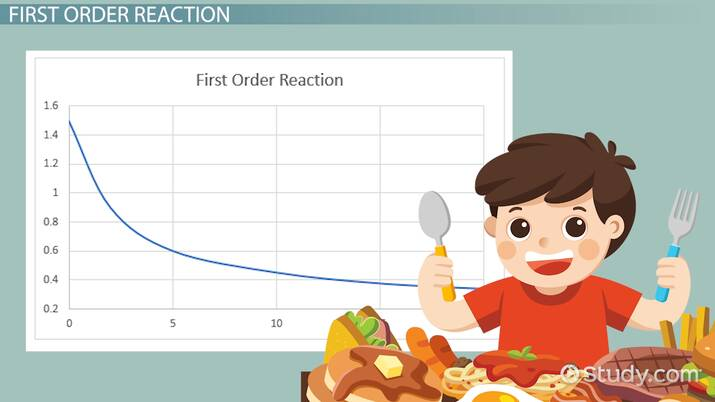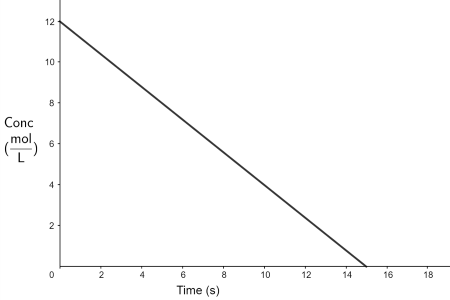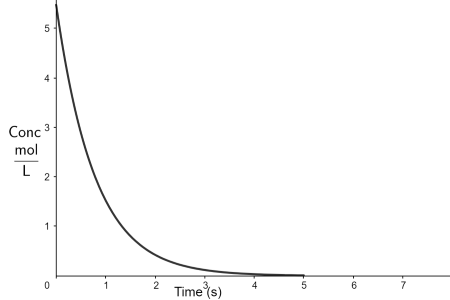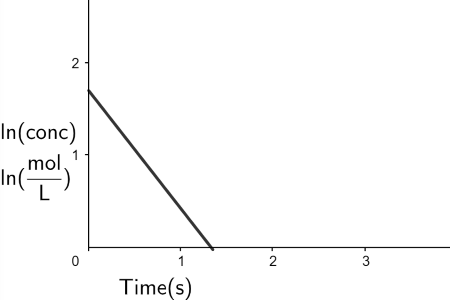# Finding Rate Constants and Order of Reactions

Kieran Gallagher, Laura Foist
• Author
Kieran Gallagher

Kieran recently graduated from Whitman College with a bachelor's degree in Chemistry and Art. During that time, he tutored high schoolers in STEM classes ranging from Geometry to AP Physics. He is set to enter a Chemistry PhD program in the fall of 2022.

• Instructor
Laura Foist

Laura has a Masters of Science in Food Science and Human Nutrition and has taught college Science.

Explore reaction order graphs. Understand how to use data from the graphs and integrated rate law to determine the order of reaction and find the rate constant. Updated: 03/07/2022

Show

## What is Order of Reaction?

Chemical reactions never happen instantaneously, it takes time for molecules to react with one another to form the products of a reaction. Each reaction has its own reaction kinetics, which are defined by its rate constant and order of the reaction. Order of reaction is defined as how the reactants' concentrations affect the rate of the reaction. The three most common are zero-, first-, and second-order reactions. The order is the sum of the exponents on each of the reactants' concentration terms in the rate law. If a reactant has a bigger exponent, its concentration affects the reaction rate more. For a general reaction:

{eq}aA + bB \rightarrow cC + dD \\\ Rate= k[A]^x[B]^y \hspace{0.5cm} Order=x+y {/eq}

Where k is the rate constant, and x and y are independent of a and b, respectively. The rate constant is a proportionality constant that generally determines how slow or fast a reaction occurs.An error occurred trying to load this video.

Try refreshing the page, or contact customer support.

Coming up next: Determining Rate Equation, Rate Law Constant & Reaction Order from Experimental Data

### You're on a roll. Keep up the good work!

Replay
Your next lesson will play in 10 seconds
• 0:04 Rate
• 0:36 Zeroth Order Reaction
• 1:53 First Order Reaction
• 2:55 Second Order Reaction
• 3:39 Lesson Summary
Save Save

Want to watch this again later?

Timeline
Autoplay
Autoplay
Speed Speed

## Zeroth Order Reaction

The simplest order of the reaction is a zero-order reaction. In zero-order reactions, the concentration of the reactants does not affect the rate of the reaction. The zero-order rate law is

{eq}Rate=k {/eq}

k is a constant value, so the rate of the reaction does not change unless other factors such as temperature and pressure change.

### Reaction Order Graph: Zeroth Order

Rate law graphs, usually called kinetics graphs, show how reactants of a reaction change over time. This graph shows a zero-order reaction.In rate order graphs, the X-axis depicts time, usually in seconds, where time=0 is the start of the reaction. The Y-axis of zero-order reaction order graphs depicts the concentration of a reactant/reactants, in mol per Liter. This graph depicts that a reactant has a concentration of 12 {eq}\frac{mol}{L} {/eq} before the reaction starts. Then the reaction starts at time=0, and 15 seconds after the reaction started, there are no reactants left. This means the reaction has run to completion. More time means less concentration for reactants because they are being transformed into products.

### How to Determine the Order of Reaction: Zeroth Order

The order of a reaction can be determined from its kinetics graph. The rate of a reaction is defined as how fast reactants are being converted into products, measured in {eq}\frac{mol}{L*s} {/eq}, and happens to be the slope of the line of the graph shown.

The line in the zero-order reaction graph is linear, which means the slope of the line does not change as the concentration of the reactant decreases, which is true for zero-order reactions.

If a reaction is zero-order, the graph of Concentration versus Time is linear.

### How to Find Rate Constant of Zeroth Order from the Graph

Once the order of the reaction is found, the rate constant can be found using that information. As far as how to find the rate constant from the graph, the integrated rate law of a zero-order reaction is used. The integrated rate-law is found by integrating Rate=k for a zero-order reaction and rearranging, which gives

{eq}[A]=-kt+[A]_0 {/eq}

Where A is the concentration at time=t, and {eq}A_0 {/eq} is the initial concentration. The formula fits nicely into the y=mx+b formula for a line, so the rate constant is the negative slope of the line plotted.

To find this, the initial and final values are taken, and the slope is found using m=change in Y / change in X. So, to do so for the zero-order reaction graph,

{eq}Rate \hspace{.02cm} Constant=-\frac{0\frac{mol}{L}-12\frac{mol}{L} }{15s-0s}=0.8 \frac{mol}{L*s} {/eq}

## First Order Reaction

A first-order reaction means that the rate of the reaction is directly related to the concentration of a reactant. If a reactant's concentration is doubled, the rate of reaction at that specific time is doubled.

A first-order reaction has a rate law of:

{eq}Rate=k[A] {/eq}

### First-Order Reaction Graph

The first-order reaction graph of the differential rate law is not as simple as the zero-order reaction graph but is still useful to compare.The graph uses the same axes as the zero-order reaction and is read in the same way. Since the rate of the reaction changes with the concentration of the reactant, there is not an easy way to glean much information from the graph.

However, if the integrated rate law is plotted with a specific axis, the reaction order and rate constant can be determined.A straight line is shown in a graph for a first-order reaction where the X-axis is time and the Y-axis is the natural log of concentration.

### How to Determine the Order of Reaction: First Order

To determine the order of reaction for first-order reactions using rate order graphs, the graph of ln(concentration) vs time is plotted. If the graph is linear, the reaction follows first-order kinetics.

### How to Find Rate Constant of First Order from the Graph

Like the zero-order example, the equation for the integrated rate law graphs is used. The integrated rate law for a first-order reaction is

{eq}ln[A]=-kt+ln[A]_0 {/eq}

To unlock this lesson you must be a Study.com Member.

#### How is the order of a reaction determined from a graph?

The reaction plotted against different Y-axes is used to determine the order of a reaction. If the line is straight when the Y-axis is concentration, the reaction is zero-order. If the line is straight when the Y-axis is ln(concentration), the reaction is first-order. If the line is straight when the Y-axis is 1/concentration, the reaction is second-order.

#### How is the rate constant k found from a graph?

Once the graph shows a straight line, the rate constant is found from the slope of the line. For zero- and first-order reactions, the rate constant is the negative slope, and for second-order reactions, the rate constant is the slope.

### Register to view this lesson

Are you a student or a teacher?